# 1 Reinforcement Learning with Videos: Combining Offline Observations with Interaction

## 1.1 目标问题

• 机器人必须能通过观察来更新策略，不需要任何的行动或者奖励；
• 人手与末端执行器视觉差异较大，自由度也不同，因此需要考虑动作空间、形态、视角、环境差异带来的变化；

## 1.2 方法

（1）问题定义

（2）方法概述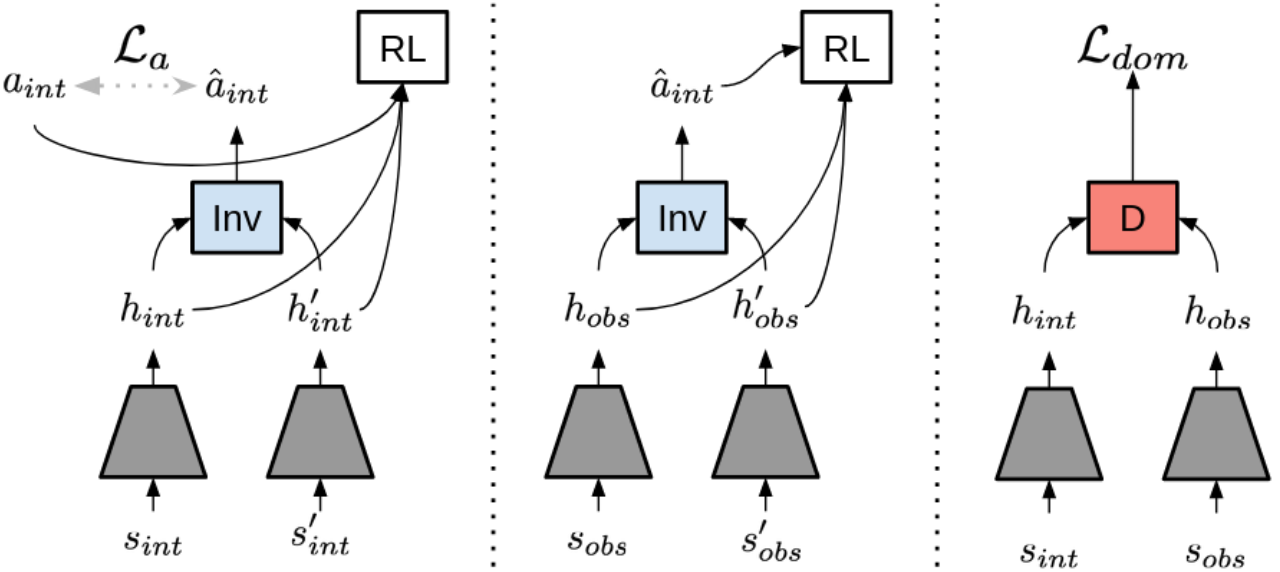• 左图：从动作条件重放池中采样数据 $(s_{int},a_{int},s_{int}‘,r_{int})$，将观测状态分别编码成特征 $h_{int},h_{int}’$，训练一个可逆的模型，来从特征中预测动作 $a_{int}$。
• 中图：将这个可逆模型用于根据观测状态特征 $h_{obs},h_{obs}'$，预测离线视频中的缺失的机器人动作 $\hat a_{int}$，将轨迹中最后一步设置为很大的奖励，前面其它步骤都设置为很小的奖励。
• 右图：使用 adversarial domain confusion(ADS)来对齐特征，最后使用离线策略强化学习算法，对于数据 $((h_{int},h_{obs}),(a_{int},\hat a_{int}),(h_{int}‘,h_{obs}’),(r_{int},\hat r_{obs}))$ 进行训练。

（3）动作预测

$$L_a(a_{int},h_{int},h_{int}‘,\theta)=||a_{int}-f_{inv}(h_{int},h_{int}’;\theta)||^2$$

（4）奖励生成

（5）域自适应

（6）联合优化

# 2 Learning Generalizable Robotic Reward Functions from “In-The-Wild” Human Videos

## 2.1 目标问题

• 人类的 wild data 和机器人的观测空间有着巨大的域变换，不管是 agent 的形态、还是场景的外观。
• 人类的动作空间和机器人的动作空间不同，可能不能很好的实现动作的映射
• 人类视频很多情况下是低质量的、有噪声的，还有着复杂的背景或视角

• 训练一个分类器预测两个视频是否完成的是同一个任务，也就是不可知域视频鉴别器（DVD）
• 训练完成后，DVD 能够将人类视频作为演示，机器人的行为作为另一个视频，输出一个分数，衡量任务成功的奖励。

## 2.2 方法

（1）Domain-Agnostic Video Discriminators

• 一个预训练视频编码器将视频 $d_i$ 编码为特征 $h_i$
• 一个全连接神经网络，预测两个视频是否完成同样的任务
• 损失函数设置见原文
• 奖励函数通过训练分类器来获得

$$R_\theta(d_i,d_j)=f_{sin}(f_{enc}(d_i),f_{enc}(d_j);\theta)$$

（2）使用 DVD 执行任务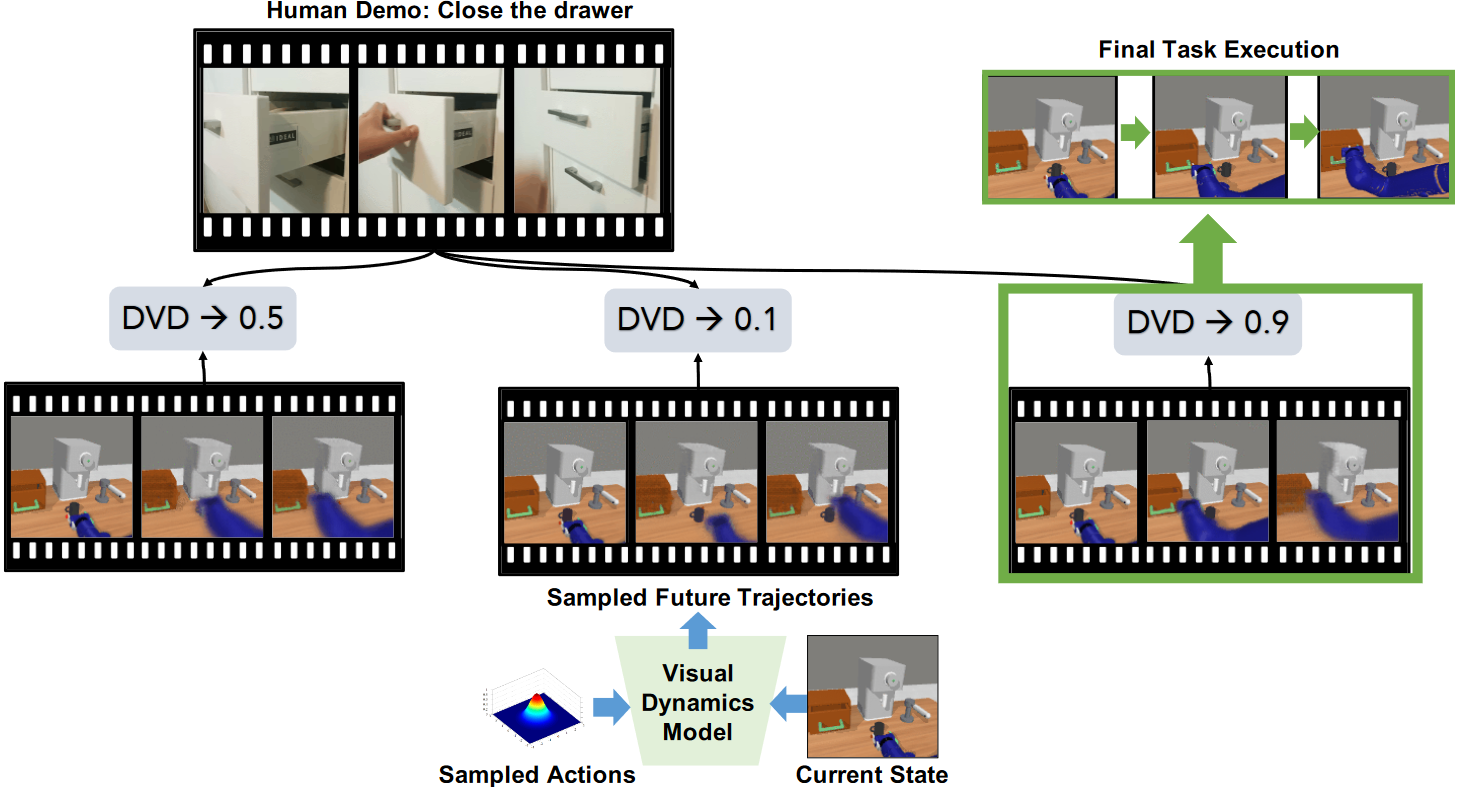1. 使用 SV2P 模型训练动作条件视频预测模型 $\rho$
2. 使用交叉熵和该动作模型 $\rho$ 选择与人类演示最相似的动作
1. 对输入图像，从动作分布中采样多个动作序列，并使用动作模型 $\rho$ 预测相应的未来轨迹
2. 将每个预测轨迹和人类演示视频，输入DVD，得到任务相似性分数
3. 执行与演示图象具有最高相似性的动作轨迹

# 3 PLAS: Latent Action Space for Offline Reinforcement Learning

## 3.2 方法

（0）原理基础-离线 RL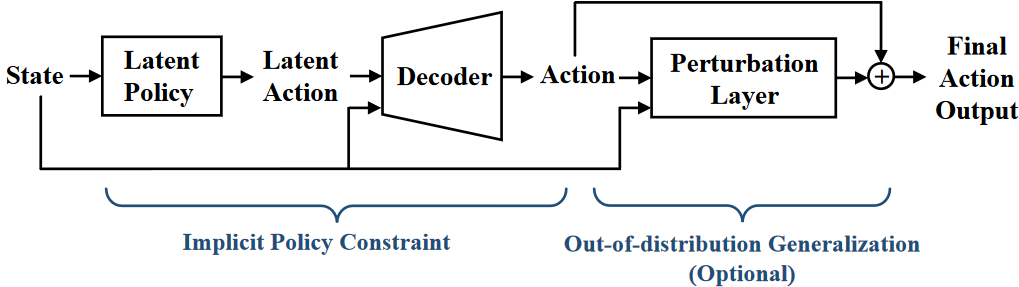（1）潜在动作空间中的策略（Policy in Latent Action Space, PLAS）

（2）泛化

# 4 Demonstration-Guided Reinforcement Learning with Efficient Exploration for Task Automation of Surgical Robot

## 4.1 目标问题

• 使用强化学习，如果不给出演示数据而仅通过探索学习，需要收集大量的数据来解决任务；
• 使用演示数据的方法，例如赋予演示数据相对于机器人探索数据更高的优先级，效率仍然低下，设置额外奖励函数的方法不仅只能针对特定环境，且容易引起局部最优；
• 使用 actor-critic 框架，通过正则化 actor 损失来衡量机器人与专家之间的行为差异，但是这种方式效率较低（尤其在初期机器人与演示差距较大情况下），且没有考虑 critic 的正则化，容易导致高估问题。

• 提出一种 actor-critic 框架，降低 critic 的高估问题，提高强化学习过程中类似专家的行动进行探索。
• 使用非参数引导传播，实现未观测状态的探索
• 在 SurRoL 手术机器人上实验验证，效果优秀，同时部署在 dVRK 上，同样表示出强大的潜力。

dVRK(da Vinci Research Kit，达芬奇手术机器人系统)

## 4.2 方法

DEX(Demonstration-guided EXploration)，演示引导探索。

（0）问题定义

off-policy，指智能体不使用当前的策略来决定行动，而是使用不同的策略来生成行为数据，从过去的经历中学到最优的行为决策方法。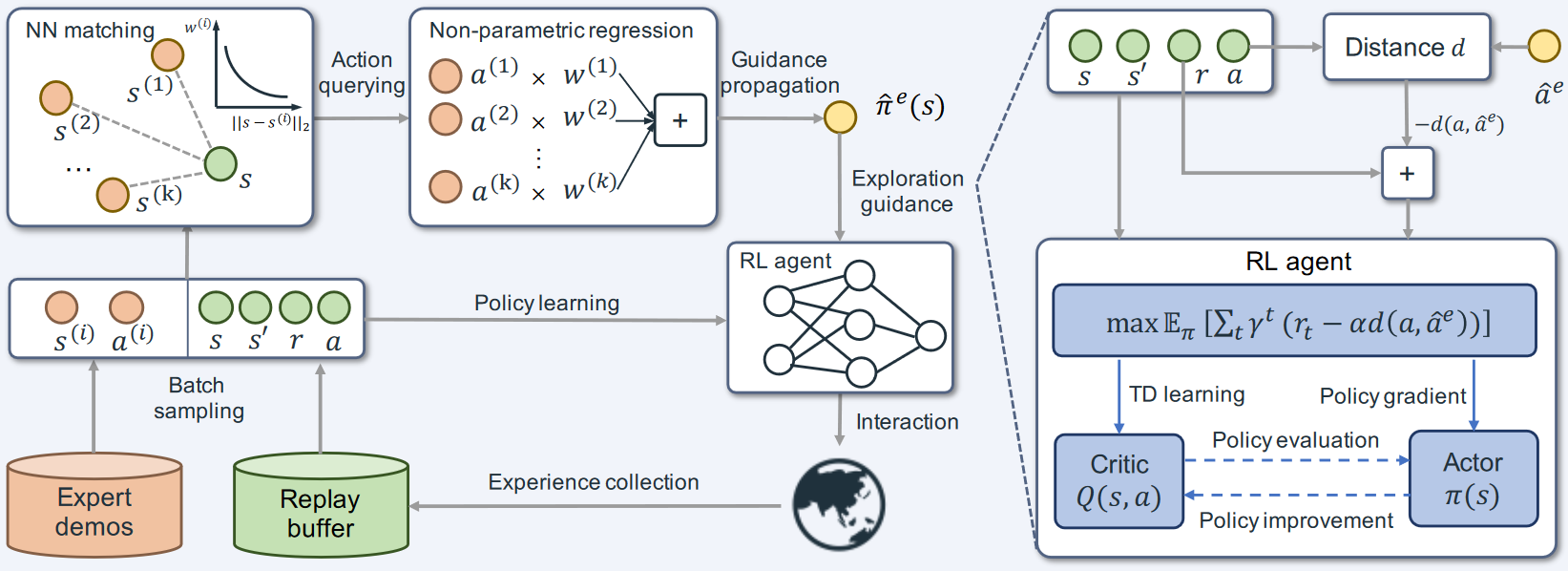• 基于 actor-critic 的策略学习模块（右下角），用于从演示数据中指导探索；
• 基于最近邻匹配和局部加权回归的非参数模块（左上角），用于将与当前状态相差过大的演示传播到为当前状态。

（1）专家引导的 actor-critic 框架

$$\max_{\pi}\mathbb{E}{\pi}\left[\sum{t=0}^{\infty}\gamma^{t}(r_{t}-\alpha d(a_{t},a_{t}^{e}))\right],a_{t}^{e}:=\pi^{e}(s_{t}),$$

（2）有限演示情况下的引导的传播

$$\hat{\pi}^e(s)=\frac{\sum_{i=1}^k\exp\left(-|s-s^{(i)}|2\right)\cdot a^{(i)}}{\sum{i=1}^k\exp\left(-|s-s^{(i)}|_2)\right)}.$$

# 5 Residual Skill Policies: Learning an Adaptable Skill-based Action Space for Reinforcement Learning for Robotics

## 5.1 目标问题

• 对所有技能进行随机抽样探索，效率极低，因为其中只有一小部分技能与当前执行的任务相关，并且这些相关的技能通常不会聚集在技能空间的同一邻域内。
• 该方法假设技能是最优的，并且下层的任务来自于技能空间的相同分布，因此学习的通用性和变化适应性有限，例如从移动方块中学习技能，则无法应对障碍物、物体变化、不同摩擦等情况。

• 状态条件技能先验：对相关技能进行采样来引导探索
• 底层残差策略：通过对技能进行细粒度的技能适应，实现任务变化的适应

## 5.2 方法

• 从现有控制器中提取技能
• 学习技能嵌入和先验技能
• 训练一个分层强化学习策略，在技能空间中使用底层残差适应性策略。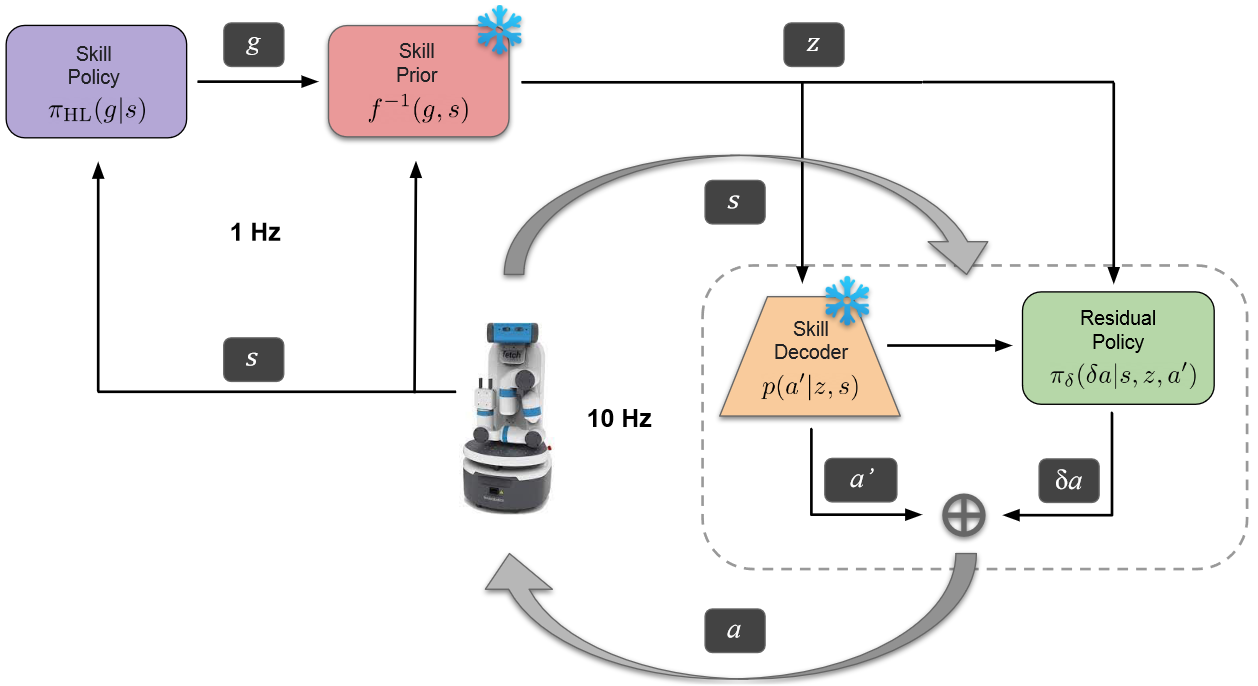（1）数据收集

（2）学习强化学习的状态条件技能空间

• 将提取的技能嵌入到潜在空间中：使用变分自动编码器 VAE 将技能 $a$ 嵌入到潜在空间中，VAE 包括编码器和解码器，编码器将完整的 state-action 序列编码为 $z$，解码器根据当前状态 $s_t$ 和技能编码 $z$ 重建动作。
• 在探索过程中采样的技能状态条件先验：学习潜在技能空间上的条件概率密度。传统的高斯密度不能处理多模态信息，本文使用 real NVP 方法，实值非体积保留变换。学习从 $Z\times S->G$ 的映射，该映射就可以从简单分布 G 变换到技能空间 Z，因此 f 就是技能先验。

（3）状态条件技能空间中的强化学习

## 5.3 总结

1. 数据收集：使用最基本的控制器生成一些基本任务轨迹（移动、抓取），然后将这些轨迹分割成固定长度的序列，每一小段包括动作和对应的状态。
2. 学习技能空间，使用变分自编码器将技能编码到潜在空间中；使用realNVP将技能潜在空间+机器人状态空间映射到简单分布空间（高斯分布），这样可以直接根据状态采样技能，称为技能先验。
3. 强化学习：使用一个高层策略网络，根据当前的状态生成一个向量，根据技能先验（与当前状态有关的技能）中选择一个技能，利用技能解码器解码成机器人动作。

# 6 Watch and Match: Supercharging Imitation with Regularized Optimal Transport

## 6.2 方法

（1）BC 预训练

BC 对应求解公式中的最大似然问题，这里的专家轨迹 $T^e$ 指的是专家演示，训练后，它能够使 $\pi^{BC}$ 模仿与演示中想对应的动作，但是如果出现未见过的状态，那么很容易会导致推理失败。

（2）在线 IRL 微调

1. 正则化微调：由于在线部署期间很容易因为错误累计导致分布偏移，本文通过基于引导 RL 和离线 RL 将 $\pi^{ROT}$ 与 BC 损失相结合来规范 $\pi^{ROT}$ 的训练，此处设置了一个 $\lambda(\pi)$ 自适应权重来控制两个损失项的贡献。
2. 柔性 Q 滤波的自适应正则化：自适应权重调整 $\lambda(\pi)$，通过比较当前策略 $\pi^{ROT}$ 和与训练策略 $\pi^{BC}$ 在一段重放缓冲区采样的一批数据的性能表现来完成。
3. 基于图像观测的考虑：对视觉观测进行数据增强，将图像输入 CNN 编码器，获得 OT 奖励的计算，减少 ROT 模仿过程中的非平稳性。